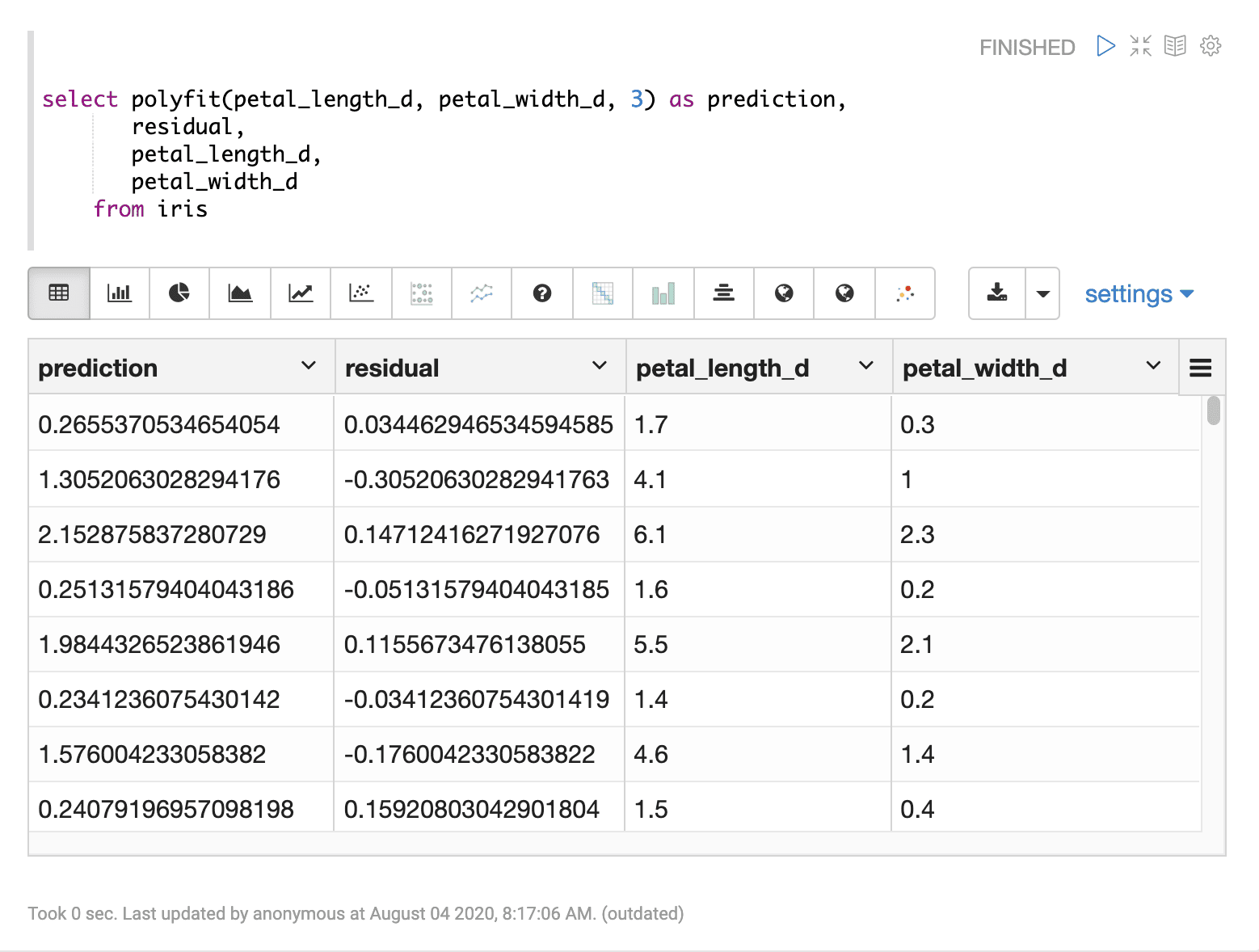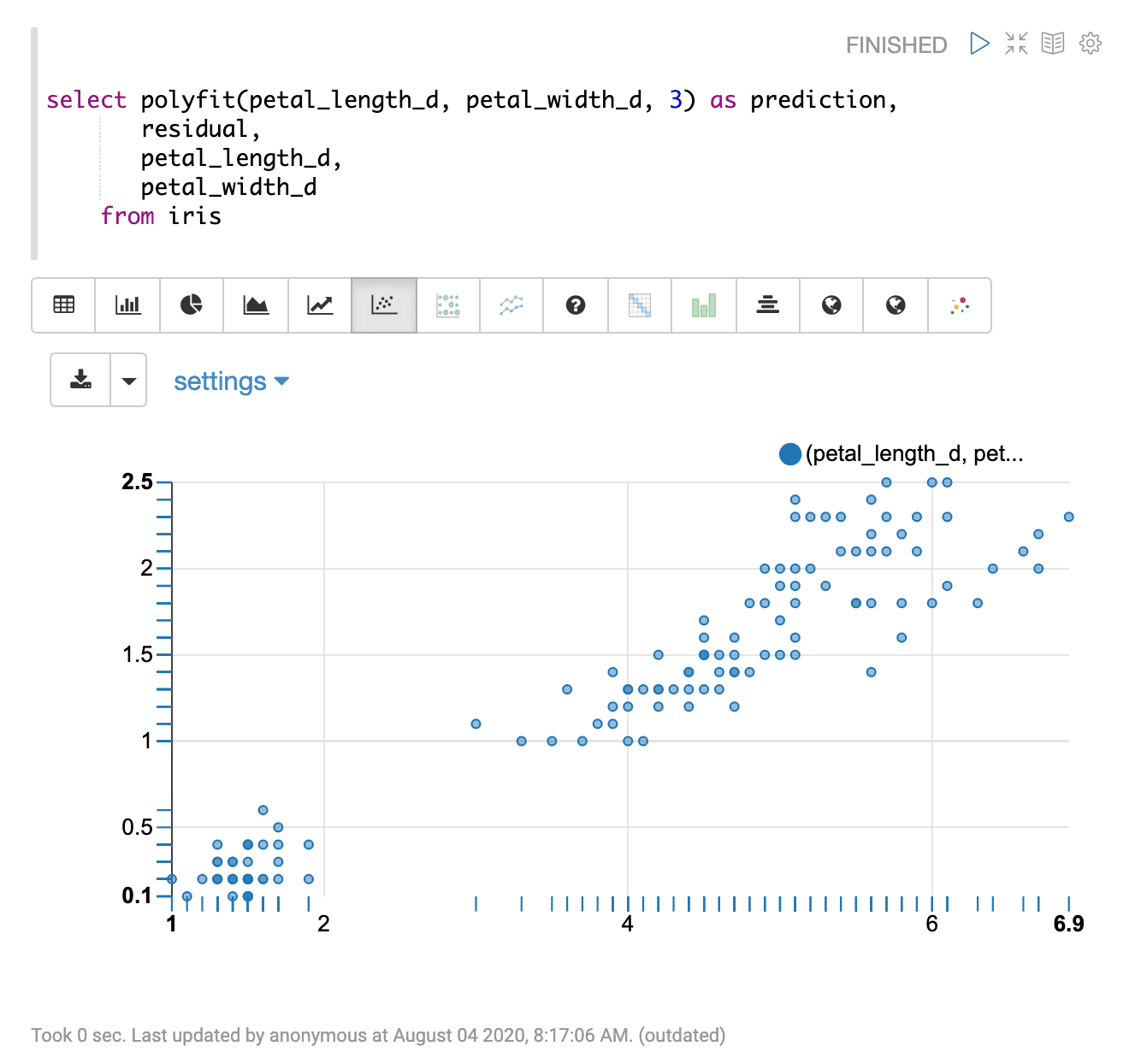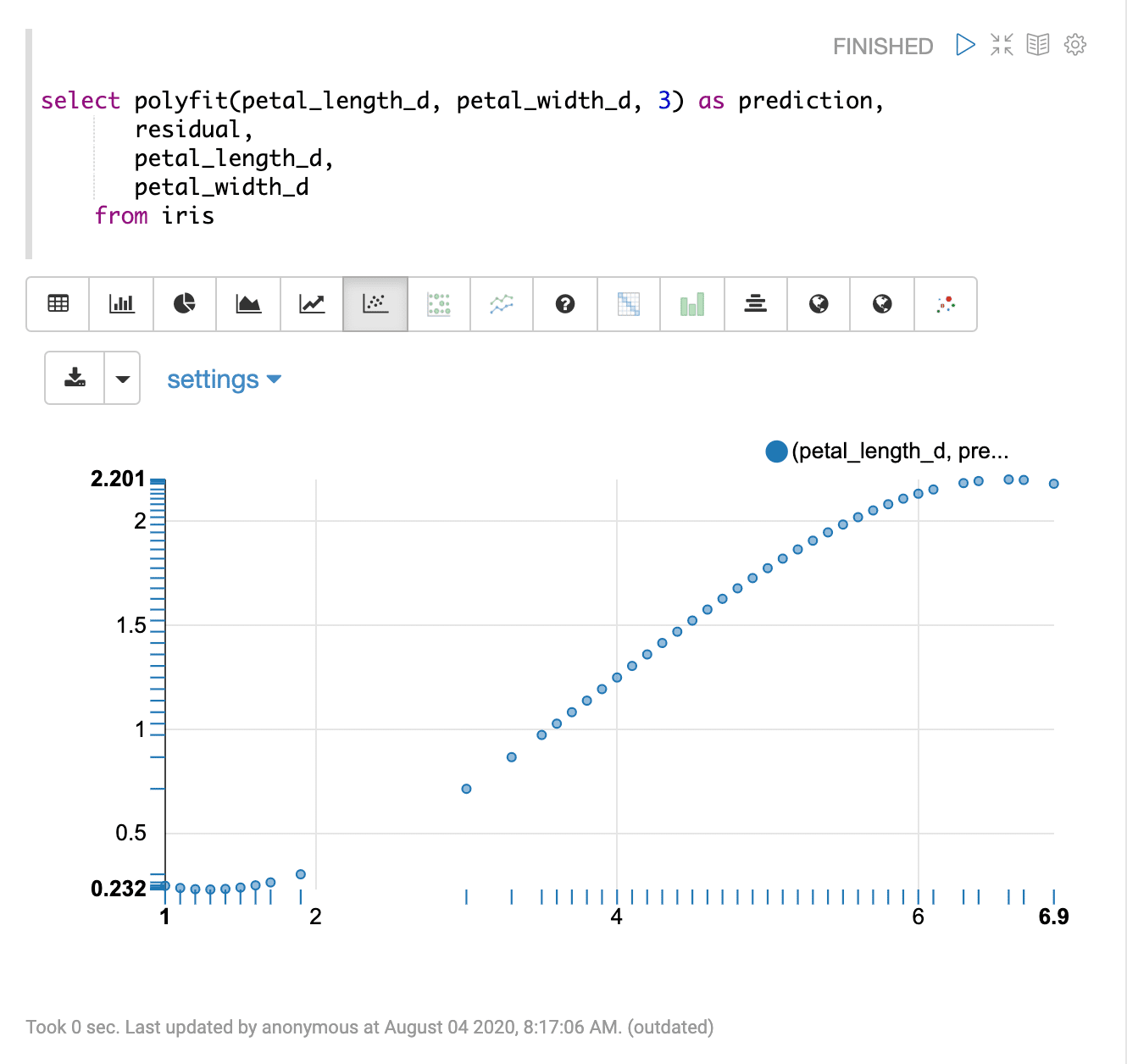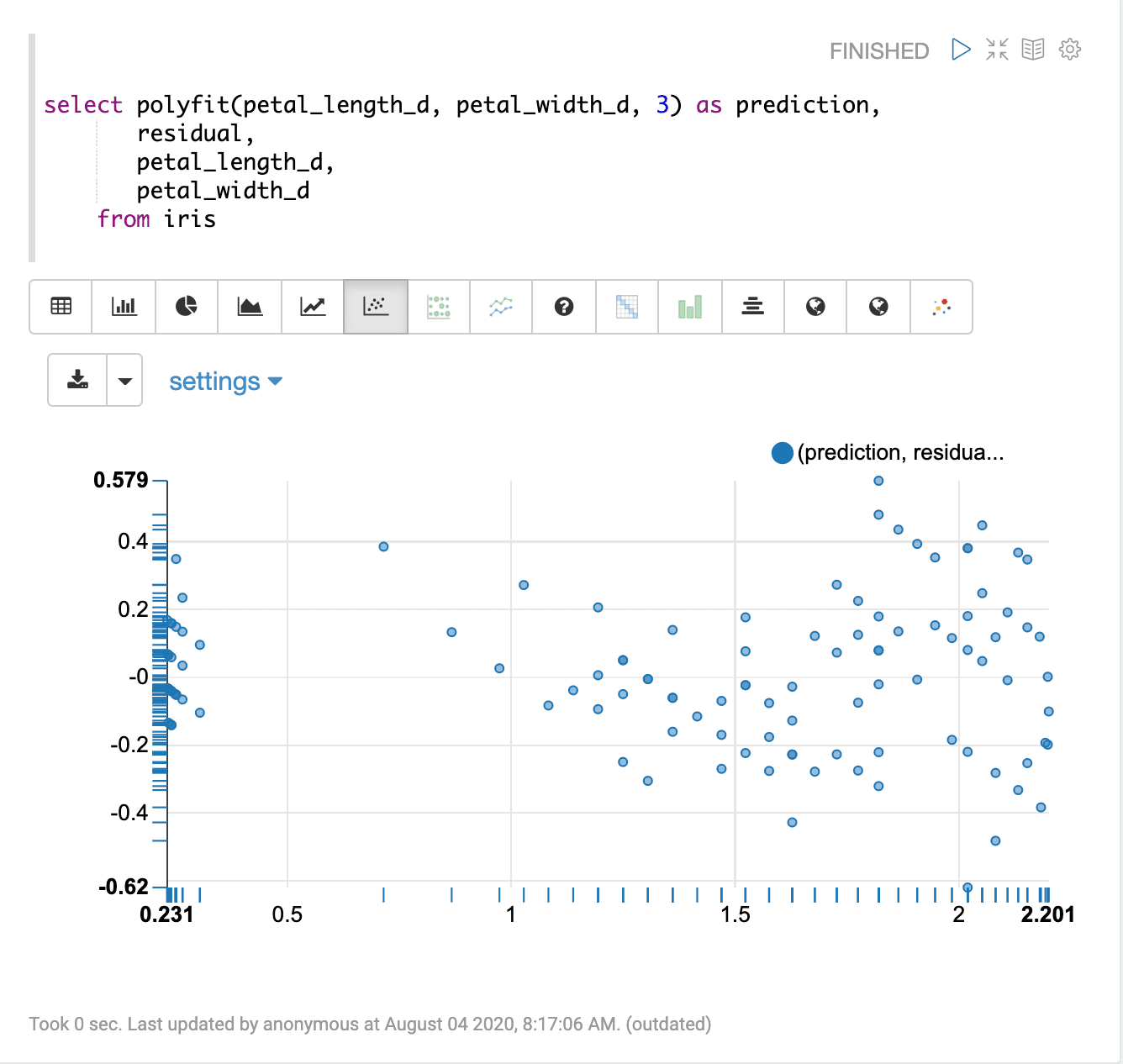# Polyfit (polyfit)

The `polyfit` function uses polynomial regression to predict a smooth, non-linear curve through a bi-variate scatter plot. The `polyfit` function takes three parameters:

1. The numeric field containing the independent (x) variable

2. The numeric field containing the dependent (y) variable

3. The integer degree of the polynomial

The degree determines the number of curves to the fitted curve. One degree polynomial performs linear regression. Typically the degree is an integer from 1 to 5.

## Sample syntax

``````select polyfit(petal_length_d, petal_width_d, 3) as prediction,
residual,
petal_length_d,
petal_width_d
from iris
limit 150``````

## Result set

The result set contains a random sample of records that match the `WHERE` clause. If no `WHERE` clause is included the random sample will be taken from the entire result set. The size of the result set can be controlled by the `LIMIT` clause. The default size, if no limit is applied, is 25000.

The `polyfit` function returns the predicted value for each record. There are three additional fields that can be selected when the `polyfit` function is used:

• `residual`: the residual value for each sample

The residual value is the samples dependent (y) value minus the predicted value. The residual represents the error of the regression prediction for each sample.

• the independent variable for each sample

• the dependent variable for each sample

Sample result set in Apache Zeppelin## Visualization

There are a number of visualizations that can flow from the regression result set.

The first visualization shown is a scatter plot with `petal_length_d` on the x-axis and `petal_width_d` on the y-axis. This can be used to visualize the relationship between the two variables in the regression analysis.The second visualization shows the `petal_length_d` variable on the x-axis and the prediction for `petal_width_d` on y-axis.The last visualization plots the predictions on the x-axis and the residual on the y-axis. This residual plot can be used to visualize the error of the regression model across the full range of predictions.